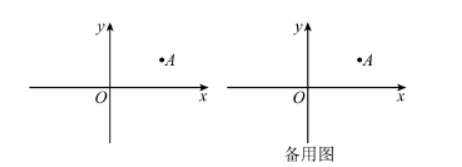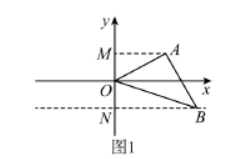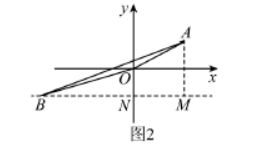(1)下列各点中, 与点 $A$ 关于 $x$ 轴斜对称的是 (只㙋序号);
① $(3,-1)$, ② $(-2,1)$, ③ $(2,-1)$, ④ $(-1,-1)$.
(2) 若点 $A$ 关于 $x$ 轴的斜对称点 $B$ 恰好落在直线 $y=k x+3 k+1$ 上, $\triangle A O B$ 的面积为 3 , 求 $k$ 的值；
(3)抛物线 $y=x^2-b x-1$ 上恰有两个点 $M 、 N$ 与点 $A$ 关于 $x$ 轴斜对称, 抛物线的顶点为 $D$, 且 $V D M N$ 为等腰直角三角形, 则 $b$ 的值为(1) 解: $\because$ 点 $A$ 的坐标为 $(2,1)$,
$\therefore$ 与点 $A$ 关于 $x$ 轴斜对称的是 $(3,-1)$ 和 $(-1,-1)$; 故答案为: ①④
（2）解：根据題意可设 $B(x,-1)$,
①如图 1，当 $x>0$ 时，\begin{aligned} & S_{\triangle A O B}=S_{\text {AMNB }}-S_{\triangle A O M}-S_{\triangle B O M} \\ & =\frac{1}{2}(2+x) \times 2-\frac{1}{2} \times 1 \times 2-\frac{1}{2} x=\frac{1}{2} x+1=3 . \end{aligned}

\begin{aligned} & \therefore B(4,-1) . \\ & \therefore 4 k+3 k+1=-1 . \end{aligned}\begin{aligned} & S_{\triangle A O B}=S_{\triangle A R M}-S_{\triangle A O N}-S_{\text {AONM }} \\ & =\frac{1}{2}(2-x) \times 2-\frac{1}{2} \times 1 \times(-x)-\frac{1}{2} \times(1+2) \times 2=-\frac{1}{2} x-1=3 . \end{aligned}

\begin{aligned} & \therefore B(-8,-1) . \\ & \therefore-8 k+3 k+1=-1 . \end{aligned}

$\therefore$ 综上所述: $k=-\frac{2}{7}$ 或 $\frac{2}{5}$.

（3）解: $\because y=x^2-b x-1=\left(x-\frac{b}{2}\right)^2-\frac{b^2+4}{4}$.
$\therefore$ 拋物线的对称轴为直线 $x=\frac{b}{2}$, 抛物线的顶点为 $\left(\frac{b}{2},-\frac{b^2+4}{4}\right)$.

$\because$ 点 $M, N$ 与点 $A$ 关于 $x$ 轴稌对称，
$\therefore$ 点 $M . N$ 的纵坐标为 -1 ,

$\therefore$ 点 $M$ 的坐标为 $(0,-1)$, 点 $N(b,-1)$,
$\because \triangle D M N$ 为等腰直角三角形。
$\therefore D M=D N$, 且 $M N^2=D M^2+D N^2=2 D M^2$,
$\therefore b^2=2\left[\left(\frac{b}{2}\right)^2+\left(-\frac{b^2+4}{4}+1\right)^2\right]$,